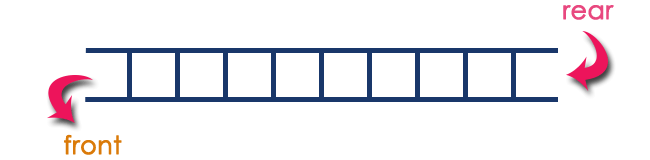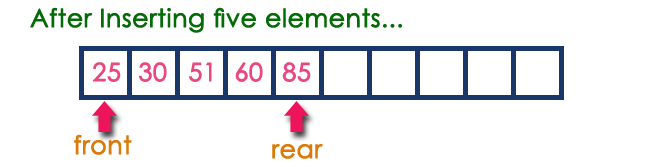The perfect place for easy learning...

×

# What is a Queue?

Queue is a linear data structure in which the insertion and deletion operations are performed at two different ends. In a queue data structure, adding and removing elements are performed at two different positions. The insertion is performed at one end and deletion is performed at another end. In a queue data structure, the insertion operation is performed at a position which is known as 'rear' and the deletion operation is performed at a position which is known as 'front'. In queue data structure, the insertion and deletion operations are performed based on FIFO (First In First Out) principle.In a queue data structure, the insertion operation is performed using a function called "enQueue()" and deletion operation is performed using a function called "deQueue()".

Queue data structure can be defined as follows...

Queue data structure is a linear data structure in which the operations are performed based on FIFO principle.

A queue data structure can also be defined as

"Queue data structure is a collection of similar data items in which insertion and deletion operations are performed based on FIFO principle".

# Example

Queue after inserting 25, 30, 51, 60 and 85.# Operations on a Queue

The following operations are performed on a queue data structure...

1. enQueue(value) - (To insert an element into the queue)
2. deQueue() - (To delete an element from the queue)
3. display() - (To display the elements of the queue)

Queue data structure can be implemented in two ways. They are as follows...

1. Using Array